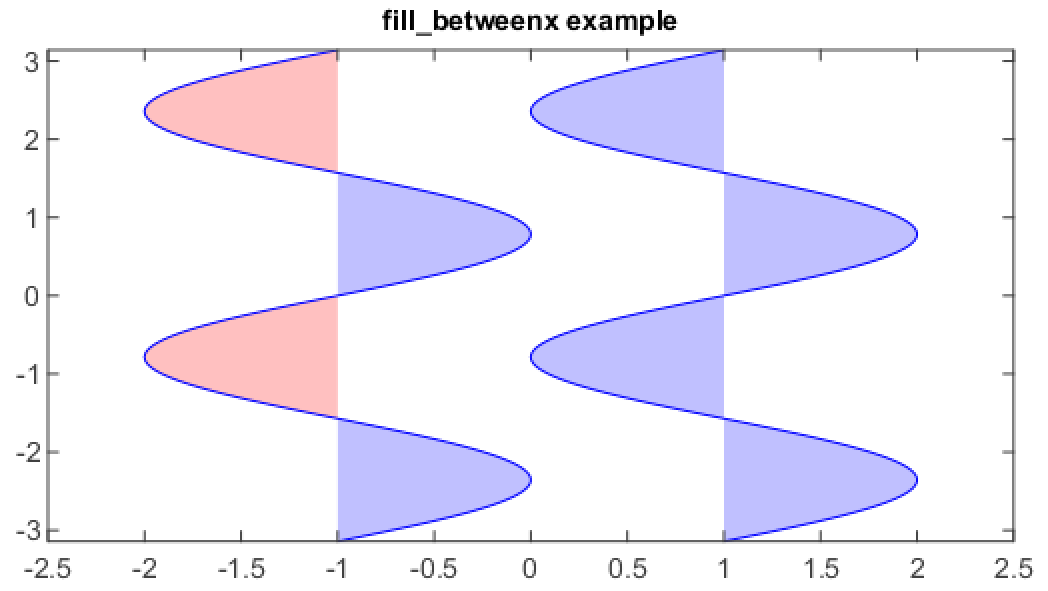# fill_betweenx¶

mipylib.plotlib.miplot.fill_betweenx(y, x1, x2=0, where=None, **kwargs)

Make filled polygons between two curves (y1 and y2) where `where==True`.

Parameters
• y – (array_like) An N-length array of the y data.

• x1 – (array_like) An N-length array (or scalar) of the x data.

• x2 – (array_like) An N-length array (or scalar) of the x data.

• where – (array_like) If None, default to fill between everywhere. If not None, it is an N-length boolean array and the fill will only happen over the regions where `where==True`.

Examples

```n = 256
y = linspace(-pi, pi, n, endpoint=True)
x = sin(2 * y)

fill_betweenx(y, 1, x+1, color='blue', alpha=.25)
plot(x+1, y, color='blue')

fill_betweenx(y, -1, x-1, (x-1) > -1, color='blue', alpha=.25)
fill_betweenx(y, -1, x-1, (x-1) < -1, color='red',  alpha=.25)
plot(x-1, y, color='blue')
ylim(-pi, pi)
xlim(-2.5, 2.5)
title('fill_betweenx example')
```